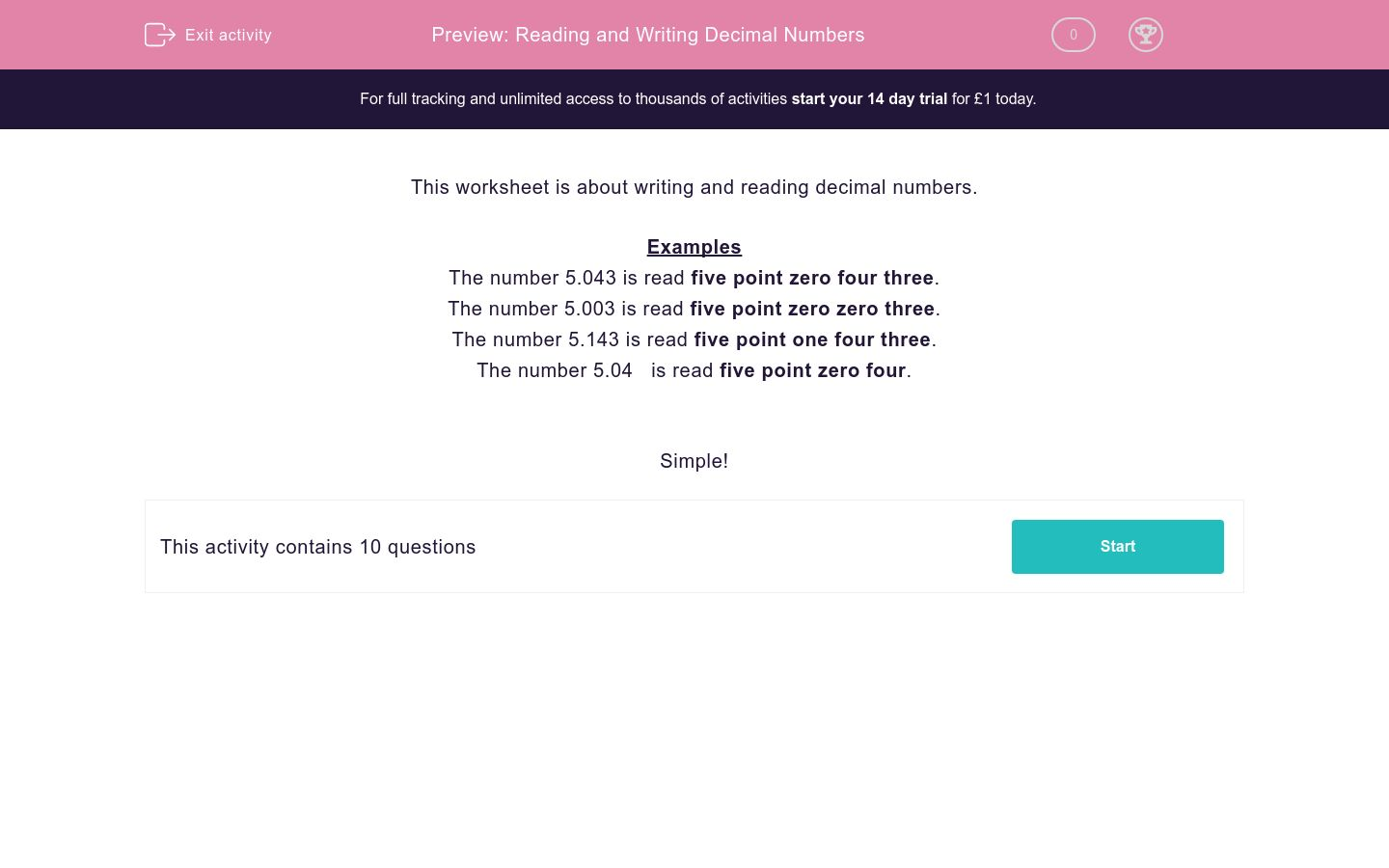# Reading and Writing Decimal Numbers

In this worksheet, students revise and practise reading and writing simple decimal numbers.Key stage:  KS 2

Curriculum topic:  Maths and Numerical Reasoning

Curriculum subtopic:  Decimals

Difficulty level:### QUESTION 1 of 10

This worksheet is about writing and reading decimal numbers.

Examples

The number 5.043 is read five point zero four three.

The number 5.003 is read five point zero zero three.

The number 5.143 is read five point one four three.

The number 5.04   is read five point zero four.

Simple!

The number 3.043 is read as...

Three point forty-three

Three point zero four three

Three zero four three

The number 5.256 is read as...

Five point two five six

Five two five six

Five point two hundred and fifty-six

The number 1.005 is read as...

one point five

one zero zero five

one point zero zero five

The number 7.728 is read as...

point seven two eight

seven point seven two eight

seven point seven twenty-eight

The number two point zero five is written as...

The number five point five zero five is written as...

The number 7.102 is read as...

seven point one two

seven one zero two

seven point one zero two

The number six point eight is written as...

6.008

6.08

6.8

The number 1.062 is read as...

one point six two

one point zero six two

one point sixty-two

The number 3.003 is read as...

Three point zero zero three

Three point zero three

Three point three

• Question 1

The number 3.043 is read as...

Three point zero four three
• Question 2

The number 5.256 is read as...

Five point two five six
• Question 3

The number 1.005 is read as...

one point zero zero five
• Question 4

The number 7.728 is read as...

seven point seven two eight
• Question 5

The number two point zero five is written as...

2.05
• Question 6

The number five point five zero five is written as...

5.505
• Question 7

The number 7.102 is read as...

seven point one zero two
• Question 8

The number six point eight is written as...

6.8
• Question 9

The number 1.062 is read as...

one point zero six two
• Question 10

The number 3.003 is read as...

Three point zero zero three
---- OR ----

Sign up for a £1 trial so you can track and measure your child's progress on this activity.

### What is EdPlace?

We're your National Curriculum aligned online education content provider helping each child succeed in English, maths and science from year 1 to GCSE. With an EdPlace account you’ll be able to track and measure progress, helping each child achieve their best. We build confidence and attainment by personalising each child’s learning at a level that suits them.

Get started#### Similar activities you may like

Start your £1 trial today.
Subscribe from £10/month.